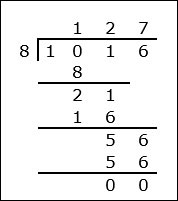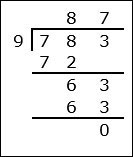# Division of a Decimal by a 1-digit Decimal

Division of a decimal by a decimal is similar to whole number division.

Rules for Decimal Division

• First we make both the decimals have the same number of decimal places if required.
• Then we divide them as if they were whole numbers ignoring the decimal points.
• The quotient so obtained is the required answer.

Consider the following examples of division of a decimal by a 1-digit decimal

Divide 101.6 ÷ 0.8

### Solution

Step 1:

101.6 has one decimal place. 0.8 has one decimal place. Here we move the decimal point to the right one place in both the numbers.

Step 2:

Now 101.6 ÷ 0.8 = 1016 ÷ 8 = 127Divide 78.3 ÷ 0.9

### Solution

Step 1:

Both 78.3 and 0.9 have one decimal place. Here we move the decimal point to the right one place in both the numbers.

Step 2:

Now 78.3 ÷ 0.9 = 783 ÷ 9 = 87